Premium

# 3.NBT.A.1 Worksheets, Workbooks, Lesson Plans, and Games

#### CCSS.maths.CONTENT.3.NBT.A.1

:
"Use place value understanding to round whole numbers to the nearest 10 or 100."

These worksheets can help students practise this Common Core State Standards skill.

## Worksheets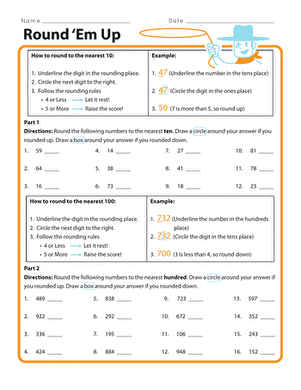Round 'Em Up
Worksheet
Round 'Em Up
Looking for a worksheet to help your kid with number rounding skills? This printable will help him with year four maths skills.
Year 4
Maths
WorksheetRounding Numbers: Subtraction
Worksheet
Rounding Numbers: Subtraction
As your second grader learns to round up or down and then complete subtraction equations, his overall maths skills will improve.
Year 3
Maths
Worksheet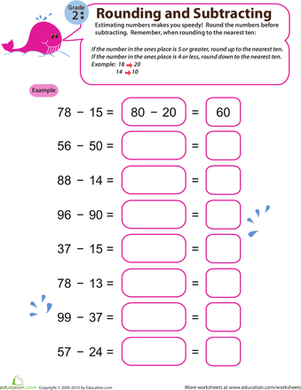Speedy Rounding and Subtracting 3
Worksheet
Speedy Rounding and Subtracting 3
With sufficient estimation practise, your second grader will gain a better understanding of numbers and significantly improve her subtraction skills!
Year 3
Maths
Worksheet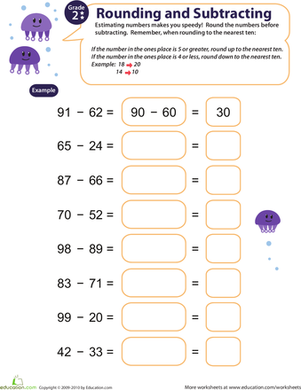Speedy Rounding and Subtracting 5
Worksheet
Speedy Rounding and Subtracting 5
With this adorable worksheet, your second grader will gain a better understanding of numbers and learn how to estimate subtraction problems quickly.
Year 3
Maths
Worksheet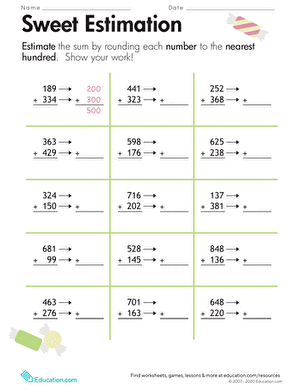Rounding: Sweet Estimation
Worksheet
Rounding: Sweet Estimation
On this year four maths worksheet, kids round to estimate the sum of two numbers, a useful strategy for checking the results of calculations.
Year 4
Maths
Worksheet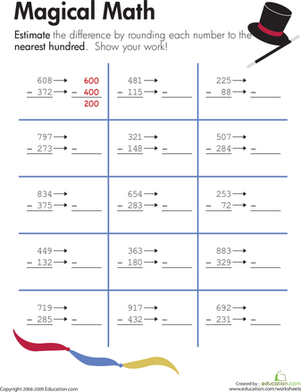Rounding: Magical maths!
Worksheet
Rounding: Magical maths!
On this year four maths worksheet, kids round to estimate the difference between two numbers, a useful strategy for checking the results of calculations.
Year 4
Maths
Worksheet

## Lesson PlansRounding Up or Down to the Nearest Ten
Lesson plan
Rounding Up or Down to the Nearest Ten
Your students will feel confident in their rounding abilities after this lesson that has them learn how to round numbers to the nearest ten both individually and with a group.
Year 4
Maths
Lesson planWhat is My Place Value?
Lesson plan
What is My Place Value?
Expand on your students' knowledge of base ten numbers with this lesson that introduces the students to the place and value of digits within a number.
Year 4
Maths
Lesson planRounding Whole Numbers
Lesson plan
Rounding Whole Numbers
It's rounding time. Help young mathematicians learn to round to the nearest ten and hundred with this teacher-approved lesson.
Year 4
Maths
Lesson plan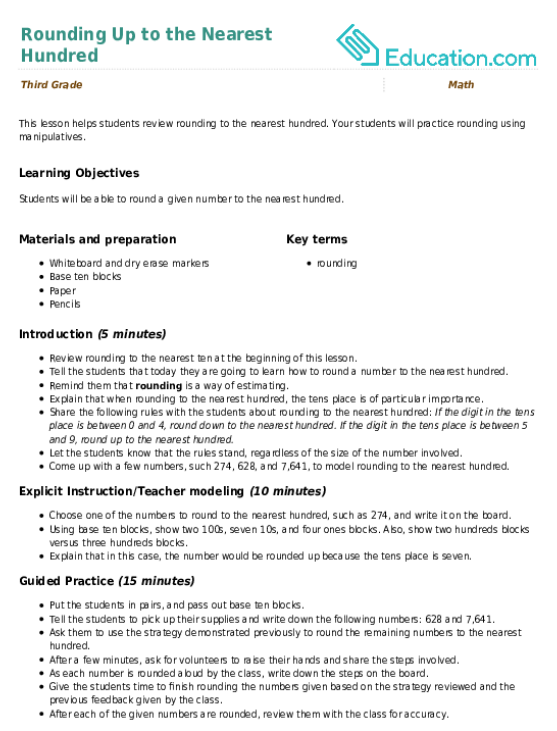Rounding Up to the Nearest Hundred
Lesson plan
Rounding Up to the Nearest Hundred
This lesson helps students review rounding to the nearest hundred. Your students will practise rounding using manipulatives.
Year 4
Maths
Lesson planRound ‘Em Up!
Lesson plan
Round ‘Em Up!
A line, an arrow, and a simple rule are all that separate students from rounding success. This lesson will give young mathematicians an engaging overview of place values and rounding.
Year 4
Maths
Lesson planRounding Around the Room!
Lesson plan
Rounding Around the Room!
Help your students understand rounding without rhymes or tricks. Students will use an open number line to round and then take part in a fun game that will get them moving around the room.
Year 4
Maths
Lesson plan

## Workbooks

No workbooks found for this common core node.

## Games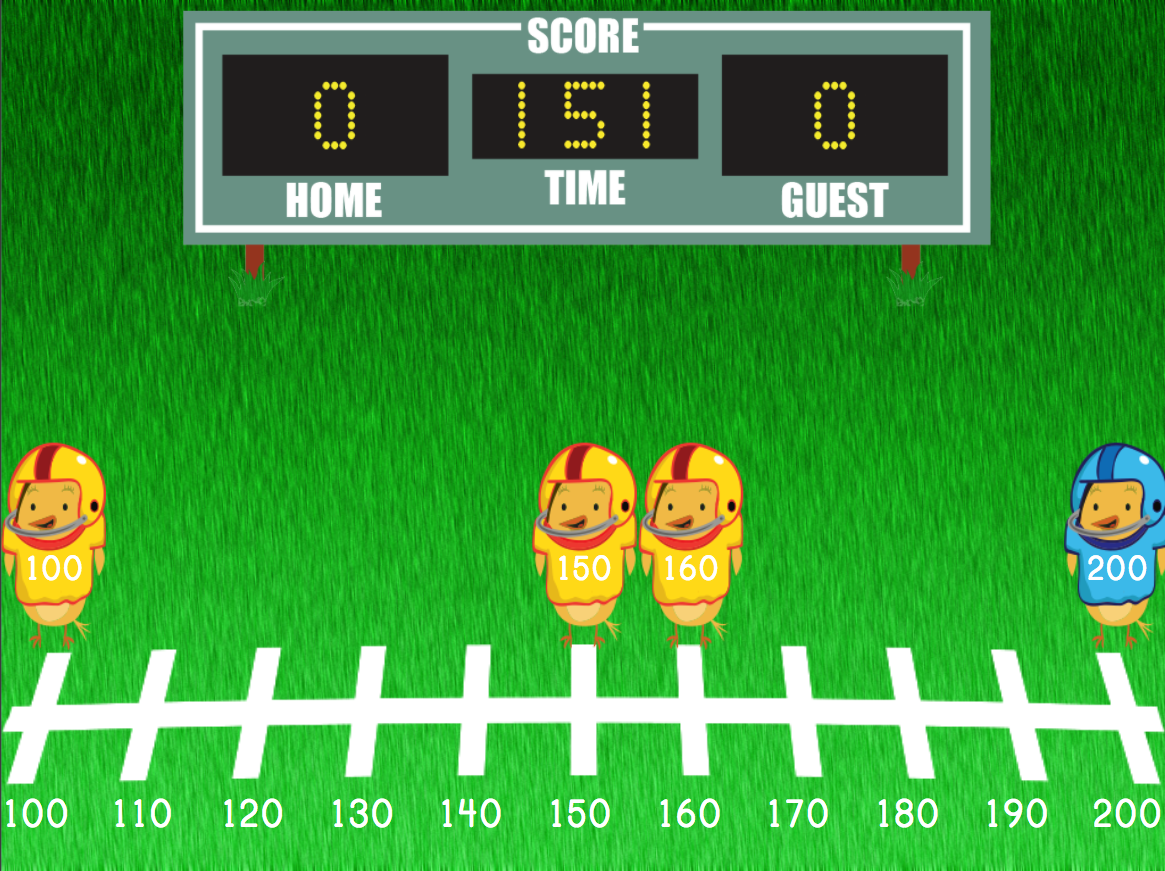Rounding Three-Digit Numbers Football
Game
Rounding Three-Digit Numbers Football
Put 'em in coach! Kids round three-digit numbers to the nearest ten or hundred in this number line game.
Year 4
Maths
Game

## Exercises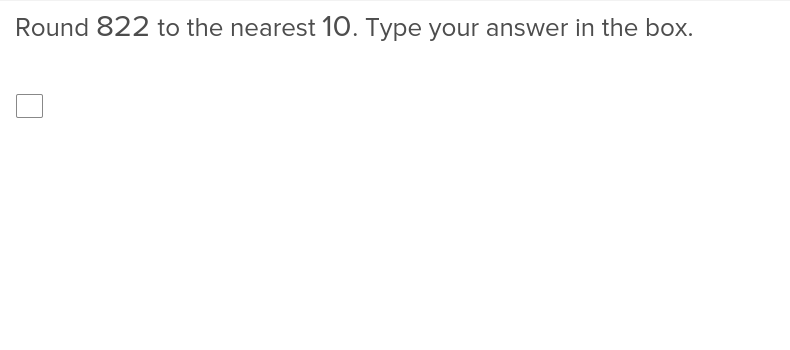Rounding to the Nearest 10
Exercise
Rounding to the Nearest 10
Teach your students how to round to the nearest 10 with this maths exercise that makes handling large numbers less cumbersome.
Year 4
Maths
Exercise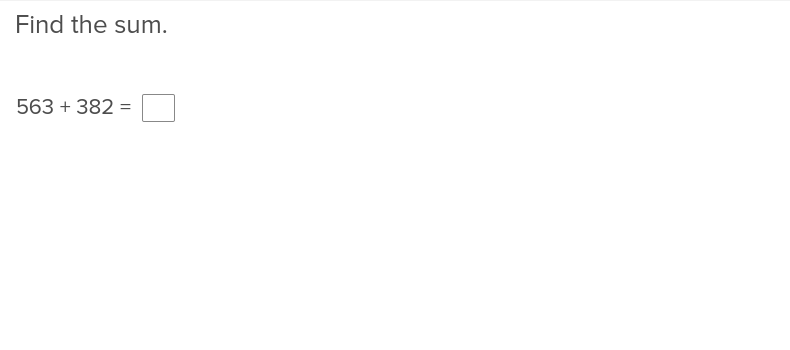Three-Digit Addition and Regrouping Numbers
Exercise
Three-Digit Addition and Regrouping Numbers
Three digit addition just got much simpler thanks to this exercise that illustrates how to regroup numbers.
Year 4
Maths
Exercise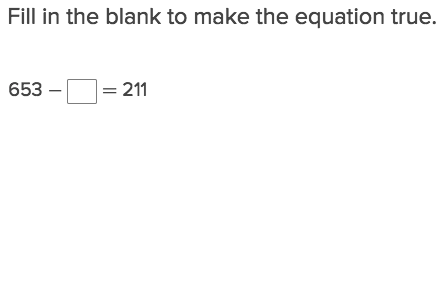Three Digit Subtraction and Missing Factors
Exercise
Three Digit Subtraction and Missing Factors
If students are confused about how to complete three digit subtraction problems with missing factors, let them work through this explanatory exercise.
Year 4
Maths
Exercise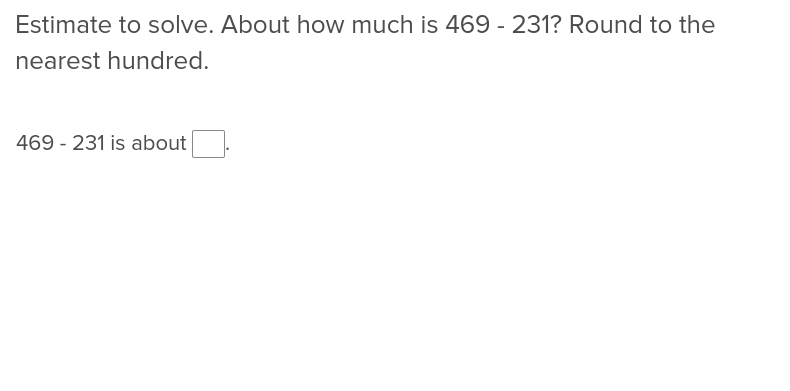Estimating to Subtract
Exercise
Estimating to Subtract
For students learning how to estimate during subtraction, this exercise will make matters much simpler.
Year 4
Maths
Exercise

### Add to collection

Create new collection

0

### New Collection>

0Items

What could we do to improve Education.com?

Please note: Use the Contact Us link at the bottom of our website for account-specific questions or issues.

What would make you love Education.com?